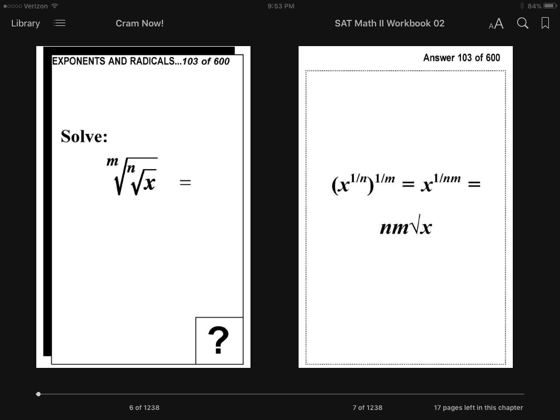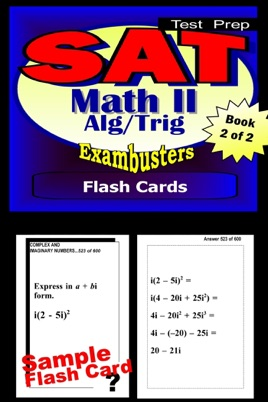• USD 4.99

## Descripción de editorial

"SAT MATH LEVEL II Prep Flashcard Workbook 2 of 2: ALGEBRA 2-TRIGONOMETRY"
500 questions and answers (ILLUSTRATED) that focus on essential advanced algebra and trigonometry concepts. Includes complementary diagrams. Essential definitions, formulas, and sample problems. Topics: Exponents and Radicals, Absolute Values and Inequalities, Polynomials, Linear Equations, Quadratic Equations, Conic Sections, Logarithms, Angles, Trigonometric Functions and Identities, Oblique Triangles, Complex and Imaginary Numbers, Area and Volume, Sequences and Series
[==================]
"SAT MATH LEVEL II Prep Flashcard Workbook 1 of 2: ALGEBRA REVIEW"
450 questions and answers. Essential definitions, formulas, concepts, and sample problems. Topics: Sets, Variables, Exponents, Properties of Numbers, Like Terms, Simple Equations, Property of Equality, Signed Numbers, Monomials, Polynomials, Advanced Equations, Verbal Problems, Factoring Polynomials, Algebraic Fractions, Equations with Several Variables, Advanced Verbal Problems, Evaluating Formulas, Simultaneous Equations, Ratio and Proportion, Variation, Quadratic Equations and Radicals, Coordinate Geometry
=============================
"EXAMBUSTERS SAT II Prep Workbooks" provide comprehensive SAT II review--one fact at a time--to prepare students to take practice SAT II tests. Each SAT II study guide focuses on fundamental concepts and definitions--a basic overview to begin studying for the SAT II exam. Up to 600 questions and answers, each volume in the SAT II series is a quick and easy, focused read. Reviewing SAT II flash cards is the first step toward more confident SAT II preparation and ultimately, higher SAT II exam scores!

•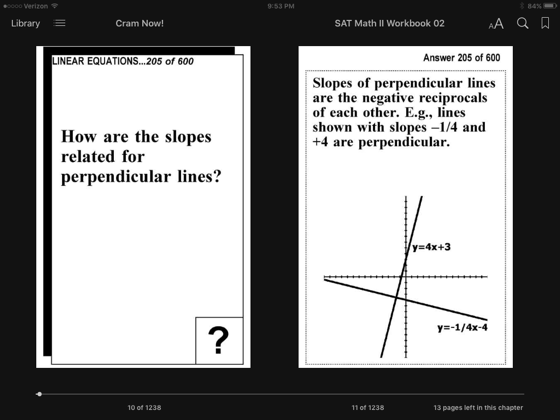•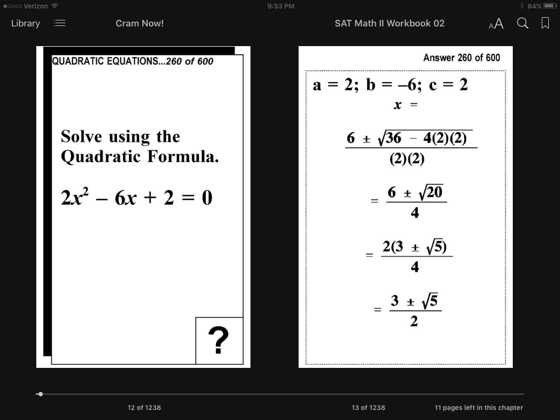•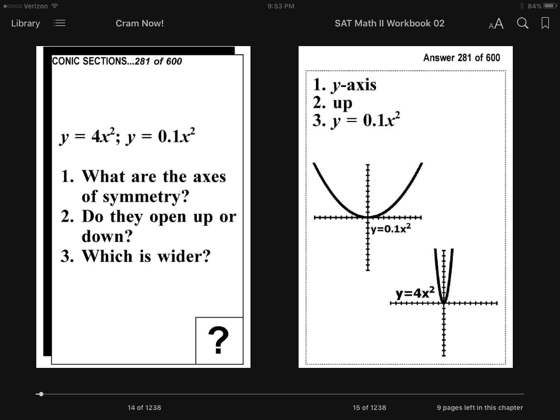•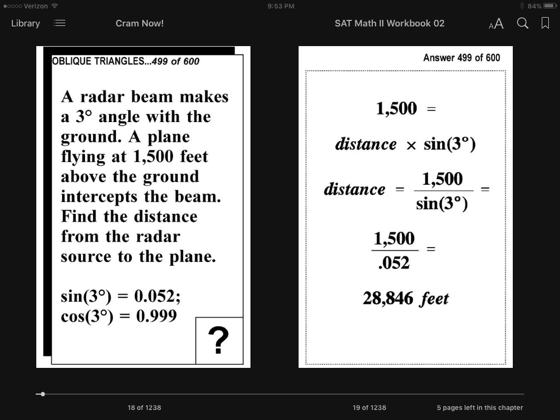•